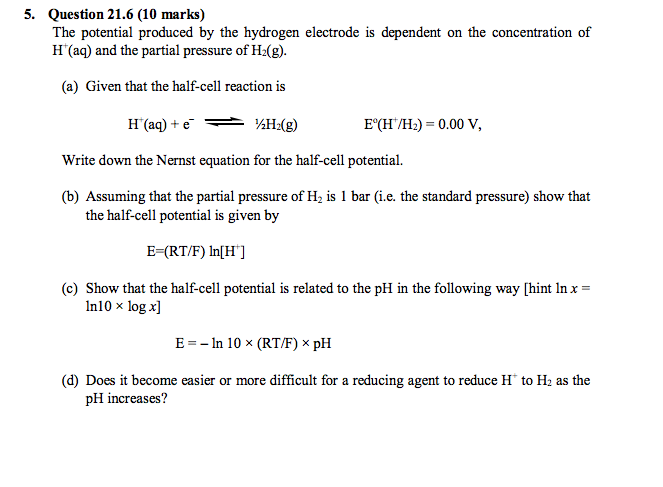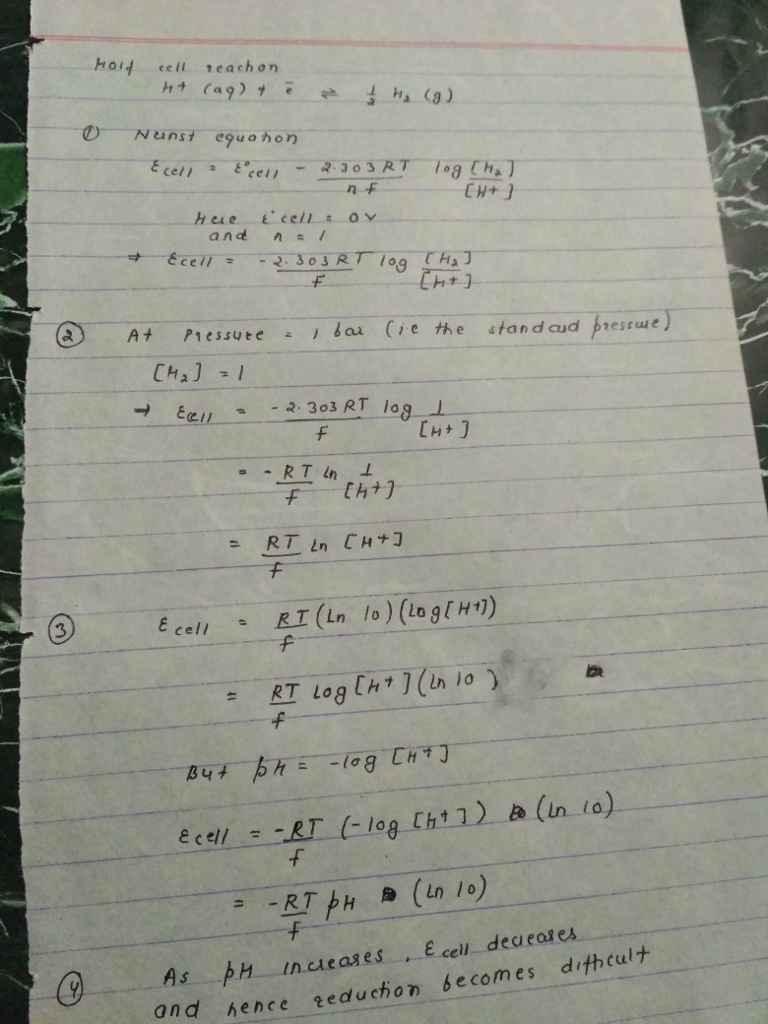# Homework Solution: The potential produced by the hydrogen electrode is dependent on the concentration of H^+…The potential produced by the hydrogen electrode is dependent on the concentration of H^+(aq) and the partial pressure of H_2(g) (a) Given that the half-cell reaction is H^+(aq) + e^- 1/2 H_2 (g) E^0 (H^+/H_2) = 0.00 V, Write down the Nernst equation for the half-cell potential. (b) Assuming that the partial pressure of H_2 is 1 bar (i.e. the standard pressure) show that the half-cell potential is given by E = (RT/F) ln[H^+] (c) Show that the half-cell potential is related to the pH in the following way [hint ln x = In10 times log x] E = -ln 10 times (RT/F) times pH (d) Does it become easier or more difficult for a reducing agent to reduce H^+ to H_2 as the pH increases?